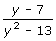Related Links

## Definition Of Expression

An Expression is a mathematical phrase that combines numbers and/or variables using mathematical operations.

An expression is a representation of a value; for example, variables and/or numerals that appear alone or in combination with operators are expressions.

### Example of Expression

##### The following are a few mathematical expressions.

5 + 6 - (3 + 2)/18
a + b - c
7 + 34
log3 (x + 1) + log3 (x - 3)### Solved Example on Expression

#### Ques: Evaluate the expression for x = 1. 2x2 + (x + 1)(x - 1) + 2(x - 3)

##### Choices:

A. - 2
B. 2
C. 0
D. 1
Correct Answer: A

### Solution:

Step 1: Substitute x = 1 in the expression 2x2 + (x + 1)(x - 1) + 2(x - 3) and then simplify.
Step 2: = 2 + 0 + (- 4)
Step 3: = - 2